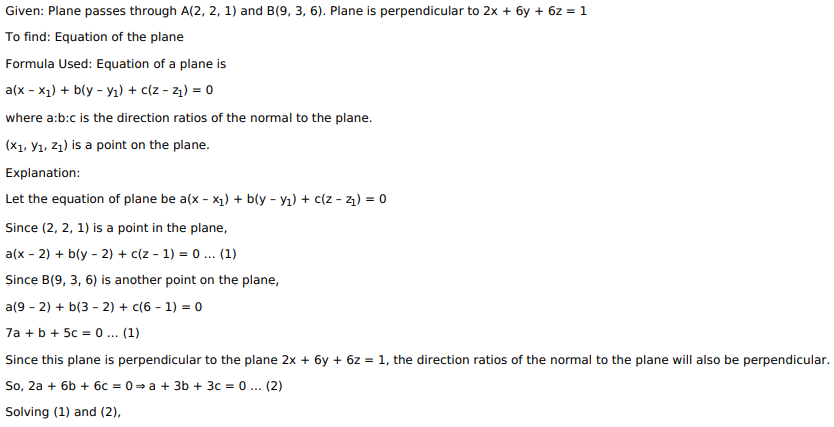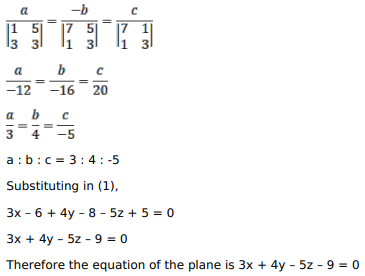# Mark against the correct answer in each of the following:Question:

Mark against the correct answer in each of the following:

The equation of the plane passing through the points $\mathrm{A}(2,2,1)$ and $\mathrm{B}(9,3,6)$ and perpendicular to the plane $2 x+6 y+6 z=1$, is

A. $x+2 y-3 z+5=0$

B. $2 x-3 y+4 z-6=0$

C. $4 x+5 y-6 z+3=0$

D. $3 x+4 y-5 z-9=0$

Solution: